# Moment

(diff) ← Older revision | Latest revision (diff) | Newer revision → (diff)

A numerical characteristic of a probability distribution. The moment of order(an integer) of a random variableis defined as the mathematical expectation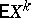, if it exists. Ifis the distribution function of the random variable, then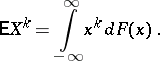(*)

For the definition of a moment in probability theory, a direct analogy is used with the corresponding idea which plays a major role in mechanics: Formula (*) is defined as the moment of a mass distribution. The first-order moment (a statistical moment in mechanics) of a random variableis the mathematical expectation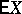. The value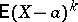is called the moment of orderrelative to,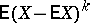is the central moment of order. The second-order central momentis called the dispersion (or variance)(the moment of inertia in mechanics). The value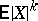is called the absolute moment of order(absolute moments are also defined for non-integral). The moments of the joint distribution of random variables(see Multi-dimensional distribution) are defined similarly: For any integers,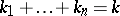, the mathematical expectation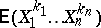is called a mixed moment of order, and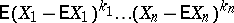is called a central mixed moment of order. The mixed moment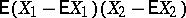is called the covariance and is one of the basic characteristics of dependency between random variables (see Correlation (in statistics)). Many properties of moments (in particular, the inequality for moments) are consequences of the fact that for any random variablethe function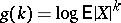is convex with respect toin each finite interval on which the function is defined;is a non-decreasing function of. The momentsandexist if and only if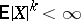. The existence of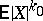implies the existence of all moments of orders. If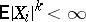for all, then the mixed moments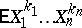exist for all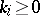,. In some cases, for the definition of moments, the so-called moment generating function is useful — the function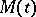with the moments of the distribution as coefficients in its power-series expansion; for integer-valued random variables this function is related to the generating functionby the relation. If, then the characteristic function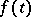of the random variablehas continuous derivatives up to orderinclusively, and the moment of orderis the coefficient ofin the expansion ofin powers of,If the characteristic function has a derivative of order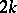at zero, then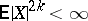.

For the connection of moments with semi-invariants see Semi-invariant. If the moments of a distribution are known, then it is possible to make some assertions about the probabilities of deviation of a random variable from its mathematical expectation in terms of inequalities; the best known are the Chebyshev inequality in probability theoryand its generalizations.

Problems of determining a probability distribution from its sequence of moments are called moment problems (cf. Moment problem). Such problems were first discussed by P.L. Chebyshev (1874) in connection with research on limit theorems. In order that the probability distribution of a random variablebe uniquely defined by its moments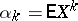it is sufficient, for example, that Carleman's condition be satisfied: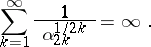A similar result even holds for moments of random vectors.

The use of moments in the proof of limit theorems is based on the following fact. Let,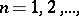be a sequence of distribution functions, all momentsof which are finite, and for each integer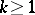let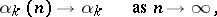whereis finite. Then there is a sequence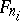that weakly converges to a distribution functionhaving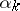as its moments. If the moments determineuniquely, then the sequenceweakly converges to. Based on this is the so-called method of moments (cf. Moments, method of (in probability theory)), used, in particular, in mathematical statistics for the study of the deviation of empirical distributions from theoretical ones, and for the statistical estimation of parameters of a distribution (on sampling moments as well as estimators for moments of a certain distribution see Empirical distribution).

The moment generating function is defined by.